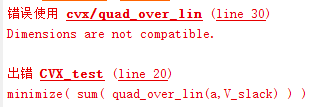# Can formulation (sum(affine/concave) < constant) be transformed to a convex expression?

I am noob in convex optimization and I have some questionsThe expression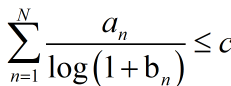seems nonconvex, where a_n and b_n are variables, c is a constant. is it possible to transform it into a convex expression??
(We know that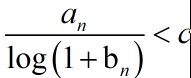can be convex since it can be transformed to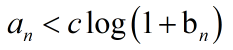.)

Anyone can give me some suggestions? thanks !!!

when you transform it to an<=c*log(1+bn) ,in order to be accepted by cvx,the left side must be convex
and the right must be concave,log(1+bn) are concave when (1+bn) are concave and >0; which have menthioned in Convex optimization in part3,不说了，急着出门。I have deleted my previous reply, because it is incorrect.

I think so. however, thanks for your kind attitude.

This is an English language forum. Thank you.

Yes, i know. As for the problem itself, I try to use English as much as i can.

In your previous reply, if the a_n is a binary variable, is it possible to use a slack variable to replace d_n*b_n ?

Section 2.8 of https://www.fico.com/en/resource-access/download/3217 shows how to linearize the product of binary variable with continuous variable.

ok, i saw this pdf in your previous answer in other posts, maybe it can help, thanks.

You can do variable substitution. Firstly, you should define x_n =\frac{a_n}{\log(1+b_n)}, \frac{a_n}{\log(1+b_{n}^{max})} \leq x_n \leq \frac{a_n}{\log(1+b_{n}^{min})}. Secondly,
you can replace \frac{a_n}{\log(1+b_n)} in the expression with x_n. Then, you have a new expression \sum_{n=1}^{N}x_n \leq c, \\ \textbf{s.t.} \frac{a_n}{\log(1+b_{n}^{max})} \leq x_n \leq \frac{a_n}{\log(1+b_{n}^{min})}. Finally, the above expression is a convex set.

that is a good idea@Yenfy I presume you are assuming a_n \ge 0.

Perhaps your formulation assumes a_n and b_n do not appear elsewhere in the model?

How are a_n and b_n recovered from x_n?

I,m sorry, it’s my fault. I thought a_n was a parameter. If a_n is a variable, my above solution is wrong.

@Yenfy If a_n were a parameter and b_n is constrained to be >= 0, the easiest thing to do in CVX would be
sum(a.*inv_pos(log(1+b))) <= c

When I use inv_pos() in CVX (not this problem.), the result usually become infeasible.,but when I comment out the constraint contains inv_pos() expression, it can be solved and the result can satify the commented consraints.， that’s very confusing.

@TomK Please provide a reproducible example, clearly showing what you say.

It seems that y cannot be a vector?
if x, y are all 1×N vectors, how to formulate sum(x(n)^2/y(n)) in CVX, N is a large constant, so I cannot formulate it as ((x(1)^2/y(1)) +(x(2)^2/y(2)) +…+(x(n)^2/y(n))) in CVX because it is too long.

y can be a vector.

quad_over_lin Sum of squares over linear.
Z=quad_over_lin(X,Y), where X is a vector and Y is a scalar, is equal to
SUM(ABS(X).^2)./Y if Y is positive, and +Inf otherwise. Y must be real.

If X is a matrix, quad_over_lin(X,Y) is a row vector containing the values
of quad_over_lin applied to each column. If X is an N-D array, the operation
is applied to the first non-singleton dimension of X.

quad_over_lin(X,Y,DIM) takes the sum along the dimension DIM of X.
A special value of DIM == 0 is accepted here, which is automatically
replaced with DIM == NDIMS(X) + 1. This has the effect of eliminating
the sum; thus quad_over_lin( X, Y, NDIMS(X) + 1 ) = ABS( X ).^2 ./ Y.

In all cases, Y must be compatible in the same sense as ./ with the squared
sum; that is, Y must be a scalar or the same size as SUM(ABS(X).^2,DIM).

Disciplined convex programming information:
quad_over_lin is convex, nonmontonic in X, and nonincreasing in Y.
Thus when used with CVX expressions, X must be convex (or affine)
and Y must be concave (or affine).

I want to minimize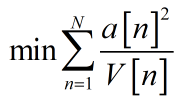This is my code:
%%%%%%%%%%%%%%%%%%%%%%%%%%
cvx_begin
% cvx_solver SeDuMi
cvx_solver SDPT3
cvx_precision low

variable a(5)
variable V_slack(5)